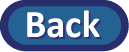## Lab Report

You are to submit your answers to the Analysis questions (k--n), and Conclusion questions (o-v) , including pasting in your applet output.

### Summary of computer skills learned in this lab:

• Creating segmented bar graphs/mosaic plot from a two-way table using technology.
• Simulating a randomization distribution to assess the significance of the difference in conditional proportions.

### Summary of statistical skills learned in this lab:

• Calculating and comparing conditional proportions.
• Approximating and interpreting a p-value from a computer simulation of random assignment.
• Approximating and interpreting a confidence interval for comparing two proportions.